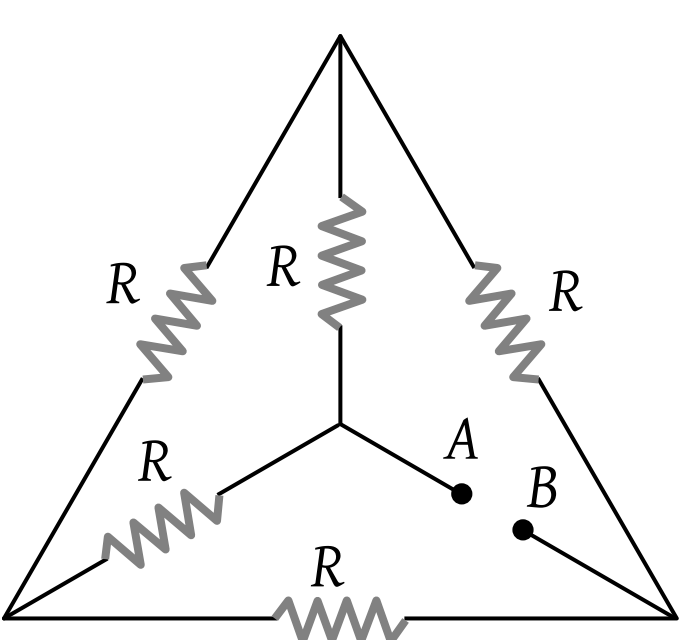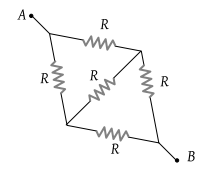NEET Questions Solved

If each of the resistance of the network shown in the figure is R, the equivalent resistance between A and B is(1) 5 R

(2) 3 R

(3) R

(4) R/2

(3) The given circuit can be redrawn as followsEquivalent resistance between A to B is R.

Difficulty Level:

• 8%
• 25%
• 65%
• 4%